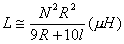# Solenoid Inductor Formulas

Solenoidal inductors are often used in high voltage, pulsed power, and power conditioning. The following diagram shows a simple single layer solenoid of length l and radius r.

The inductance of a solenoid can be found from the following formulas:Equation for the Inductance of a Single Layer Solenoid Based on the Radius and Length (in Inches) and Number of Turns

where N is the number of turns, R is the radius of the solenoid (in inches), and l is the length of the solenoid (in inches).Equation for the Inductance of a Single Layer Solenoid Based on the Radius and Length (in Cm) and Number of Turns

where N is the number of turns, R is the radius of the solenoid (in cm), and l is the length of the solenoid (in cm).

The link below will take the user to a web page with a simple calculator to determine the inductance of a given solenoid configuration (based on the number of turns, coil radius, and height or length) using this first equation. It should also be noted that there are a number of other useful calculators on this same overall website.

Send consulting inquiries, comments, and suggestions to richard.ness@nessengr.com